# Quiz 12: Replacement Decisions

Business

Given information: • Price of truck is \$12,000. • Salvage value is \$3,000. • First year operating and maintenance cost is \$2,500. • Operating and maintenance cost increases by \$500 after 2 nd year. • Interest rate is 10%. First year net cash flow: Net cash flow can be calculated by using the following formula.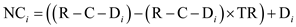…… (1)Where,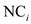= net cash flow of the i th year R = annual operating revenue C = annual operating cost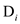= depreciation of the i th year TR = tax rate Substitute the respective values in Equation (1) to calculate the first year net cash flow of machine a.Hence, the first year net cash flow is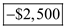. Net cash flow for all the year: Hence, similar calculation is done in Table-1 to obtain the net cash flow by using the Equation (1). Final year net cash flow is the sum of annual cash flow and salvage value.Table-1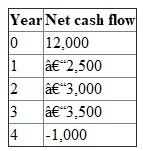Present equivalent worth: Present equivalent worth can be calculated by using the following formula: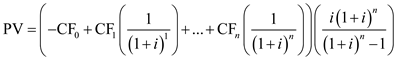…… (2)Where, i = interest. n = time period.PV = present value.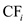= cash flow of i th year Substitute the respective values in Equation (4) to calculate the present equivalent value.Hence, the annual equivalent value is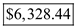.

Given information: Table-1 shows the details of the cash flow of two machines. Table-1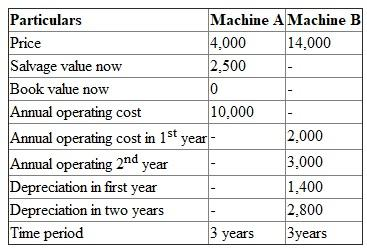• Income tax rate is 34 percent. • Any gain will be taxed 34 percent. • Interest rate is 15%. Tax gain of Machine A: Tax gain can be calculated by using the following formula: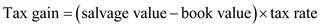…… (1)Substitute the respective values in Equation (1) to calculate the tax gain of machine a.Tax gain of machine A is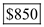. Hence, option b is correct.

Given information: • Price of printing machine is \$20,000. • Salvage value is \$5,000. • Repair cost is \$2,500. • Operating cost is \$8,000 per year. • Salvage value reduced as \$2,500 for remaining life time.• Present book value is \$14,693. • Market value is \$10,000. • Life time is 5 years. Relevant values for the analysis: Market value is the most relevant for the analysis of the defender because this value can trade the asset at present. Book value is also very important since the loss or gain of the defender can be calculated from the book value.

There is no answer for this question

There is no answer for this question

There is no answer for this question

There is no answer for this question

There is no answer for this question

There is no answer for this question

There is no answer for this question